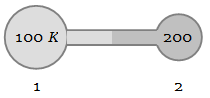At what temperature volume of an ideal gas at 0°C becomes triple :

1. 546°C

2. 182°C

3. 819°C

4. 646°C

Concept Questions :-

Types of velocity
High Yielding Test Series + Question Bank - NEET 2020

Difficulty Level:

If an ideal gas has volume V at 27°C and it is heated at a constant pressure so that its volume becomes 1.5V. Then the value of final temperature will be

1. 600°C

2. 177°C

3. 817°C

4. None of these

Concept Questions :-

Ideal gas
High Yielding Test Series + Question Bank - NEET 2020

Difficulty Level:

A tyre kept outside in sunlight bursts off after sometime because of :

1. Increase in pressure

2. Increases in volume

3. Both (a) and (b)

4. None of these

Concept Questions :-

Specific heat
High Yielding Test Series + Question Bank - NEET 2020

Difficulty Level:

In Boyle's law what remains constant :

1. $\mathrm{PV}$

2. $\mathrm{TV}$

3. $\frac{\mathrm{V}}{\mathrm{T}}$

4. $\frac{\mathrm{P}}{\mathrm{T}}$

Concept Questions :-

Ideal gas
High Yielding Test Series + Question Bank - NEET 2020

Difficulty Level:

Equation of gas in terms of pressure (P), absolute temperature (T) and density (d) is :

1. $\frac{{\mathrm{P}}_{1}}{{\mathrm{T}}_{1}{\mathrm{d}}_{1}}=\frac{{\mathrm{P}}_{2}}{{\mathrm{T}}_{2}{\mathrm{d}}_{2}}$

2. $\frac{{\mathrm{P}}_{1}{\mathrm{T}}_{1}}{{\mathrm{d}}_{1}}=\frac{{\mathrm{P}}_{2}{\mathrm{T}}_{2}}{{\mathrm{d}}_{2}}$

3. $\frac{{\mathrm{P}}_{1}{\mathrm{d}}_{2}}{{\mathrm{T}}_{1}}=\frac{{\mathrm{P}}_{2}{\mathrm{d}}_{1}}{{\mathrm{T}}_{1}}$

4. $\frac{{\mathrm{P}}_{1}{\mathrm{d}}_{1}}{{\mathrm{T}}_{1}}=\frac{{\mathrm{P}}_{2}{\mathrm{d}}_{2}}{{\mathrm{T}}_{2}}$

Concept Questions :-

Ideal gas
High Yielding Test Series + Question Bank - NEET 2020

Difficulty Level:

The figure shows two flasks connected to each other. The volume of the flask 1 is twice that of flask 2. The system is filled with an ideal gas at temperature 100 K and 200 K respectively. If the mass of the gas in 1 be m then what is the mass of the gas in flask 21. $\mathrm{m}$

2. $\frac{\mathrm{m}}{2}$

3. $\frac{\mathrm{m}}{4}$

4. $\frac{\mathrm{m}}{8}$

Concept Questions :-

Types of velocity
High Yielding Test Series + Question Bank - NEET 2020

Difficulty Level:

The absolute temperature of a gas is determined by

1. The average momentum of the molecules

2. The velocity of sound in the gas

3. The number of molecules in the gas

4. The mean square velocity of the molecules

Concept Questions :-

Types of velocity
High Yielding Test Series + Question Bank - NEET 2020

Difficulty Level:

At what temperature is the root mean square velocity of gaseous hydrogen molecules is equal to that of oxygen molecules at 47°C

1.  20 K

2.  80 K

3.  – 73 K

4.  3 K

Concept Questions :-

Types of velocity
High Yielding Test Series + Question Bank - NEET 2020

Difficulty Level:

The root mean square velocity of a gas molecule of mass m at a given temperature is proportional to

1. ${\mathrm{m}}^{0}$

2. $\mathrm{m}$

3. $\sqrt{\mathrm{m}}$

4. $\frac{1}{\sqrt{\mathrm{m}}}$

Concept Questions :-

Types of velocity
High Yielding Test Series + Question Bank - NEET 2020

Difficulty Level:

At room temperature, the r.m.s. speed of the molecules of certain diatomic gas is found to be 1930 m/s. The gas is :

1. ${\mathrm{H}}_{2}$

2. ${\mathrm{F}}_{2}$

3. ${\mathrm{O}}_{2}$

4. ${\mathrm{Cl}}_{2}$

Concept Questions :-

Types of velocity